Function Repository Resource:

# JacobianDeterminant

Compute the Jacobian determinant of a vector function with respect to a list of variables

Contributed by: Wolfram|Alpha Math Team
 ResourceFunction["JacobianDeterminant"][{f1,f2,…,fn},{x1,x2,…,xm}] computes the Jacobian matrix of the vector function {f1,f2,…,fn} with respect to the variables xi.

## Details and Options

The Jacobian matrix J of a vector mapping {x1,x2,,xm}{f1,f2,,fn} is defined as the matrix with components Jij =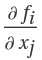. ResourceFunction["JacobianDeterminant"] returns the determinant of this matrix.

## Examples

### Basic Examples (3)

Compute the Jacobian determinant of a symbolic vector expression in two dimensions:

 In:=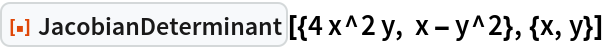Out=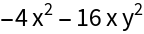Compute the Jacobian determinant of mappings in three dimensions:

 In:=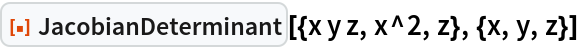Out=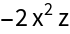In:=Out=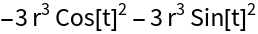Compute the Jacobian determinant of mapping between polar and Cartesian coordinates in the plane:

 In:=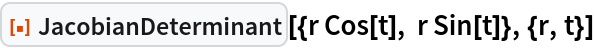Out=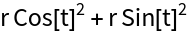### Properties and Relations (2)

Compute the Jacobian determinant of an expression:

 In:=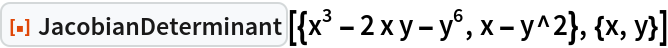Out=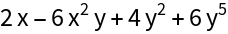Compare this to the result obtained by taking the determinant of ResourceFunction["JacobianMatrix"] of the same expression:

 In:=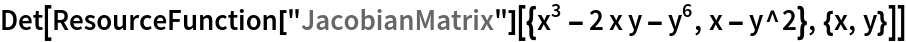Out=## Publisher

Wolfram|Alpha Math Team

## Version History

• 6.0.0 – 23 March 2023
• 5.1.0 – 12 May 2021
• 5.0.0 – 18 February 2020
• 4.0.0 – 12 February 2020
• 2.0.0 – 06 September 2019
• 1.0.0 – 05 August 2019